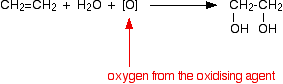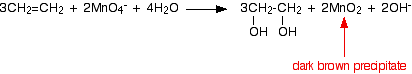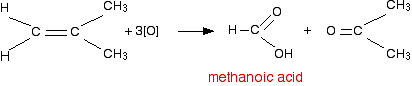# Oxidation of Alkenes with Potassium Manganate

$$\newcommand{\vecs}{\overset { \rightharpoonup} {\mathbf{#1}} }$$ $$\newcommand{\vecd}{\overset{-\!-\!\rightharpoonup}{\vphantom{a}\smash {#1}}}$$$$\newcommand{\id}{\mathrm{id}}$$ $$\newcommand{\Span}{\mathrm{span}}$$ $$\newcommand{\kernel}{\mathrm{null}\,}$$ $$\newcommand{\range}{\mathrm{range}\,}$$ $$\newcommand{\RealPart}{\mathrm{Re}}$$ $$\newcommand{\ImaginaryPart}{\mathrm{Im}}$$ $$\newcommand{\Argument}{\mathrm{Arg}}$$ $$\newcommand{\norm}{\| #1 \|}$$ $$\newcommand{\inner}{\langle #1, #2 \rangle}$$ $$\newcommand{\Span}{\mathrm{span}}$$ $$\newcommand{\id}{\mathrm{id}}$$ $$\newcommand{\Span}{\mathrm{span}}$$ $$\newcommand{\kernel}{\mathrm{null}\,}$$ $$\newcommand{\range}{\mathrm{range}\,}$$ $$\newcommand{\RealPart}{\mathrm{Re}}$$ $$\newcommand{\ImaginaryPart}{\mathrm{Im}}$$ $$\newcommand{\Argument}{\mathrm{Arg}}$$ $$\newcommand{\norm}{\| #1 \|}$$ $$\newcommand{\inner}{\langle #1, #2 \rangle}$$ $$\newcommand{\Span}{\mathrm{span}}$$$$\newcommand{\AA}{\unicode[.8,0]{x212B}}$$

The carbon-carbon double bonds in alkenes such as ethene react with potassium manganate(VII) solution (potassium permanganate solution).

## Oxidation of alkenes with cold dilute potassium manganate(VII) solution

Alkenes react with potassium manganate(VII) solution in the cold. The colorchange depends on whether the potassium manganate(VII) is used under acidic or alkaline conditions.

• If the potassium manganate(VII) solution is acidified with dilute sulfuric acid, the purple solution becomes colorless.
• If the potassium manganate(VII) solution is made slightly alkaline (often by adding sodium carbonate solution), the purple solution first becomes dark green and then produces a dark brown precipitate.

## Chemistry of the Reaction

We'll look at the reaction with ethene. Other alkenes react in just the same way. Manganate(VII) ions are a strong oxidizing agent, and in the first instance oxidize ethene to ethane-1,2-diol (old name: ethylene glycol). Looking at the equation purely from the point of view of the organic reaction:This type of equation is quite commonly used in organic chemistry. Oxygen written in square brackets is taken to mean "oxygen from an oxidizing agent". The reason for this is that a more normal equation tends to obscure the organic change in a mass of other detail - as you will find below!

The full equation depends on the conditions.

• Under acidic conditions, the manganate(VII) ions are reduced to manganese(II) ions.• Under alkaline conditions, the manganate(VII) ions are first reduced to green manganate(VI) ions . . .. . . and then further to dark brown solid manganese(IV) oxide (manganese dioxide).This last reaction is also the one you would get if the reaction was done under neutral conditions. You will notice that there are neither hydrogen ions nor hydroxide ions on the left-hand side of the equation.

You might possibly remember that further up the page it says that potassium manganate(VII) is often made slightly alkaline by adding sodium carbonate solution. Where are the hydroxide ions in this? Carbonate ions react with water to some extent to produce hydrogencarbonate ions and hydroxide ions.

$CO_3^{2-} + H_2O \rightleftharpoons HCO_3^- + OH^-$

It is the presence of these hydroxide ions that gives sodium carbonate solution its pH in the 10 - 11 region.

## Using the reaction to test for carbon-carbon double bonds

If an organic compound reacts with dilute alkaline potassium manganate(VII) solution in the cold to give a green solution followed by a dark brown precipitate, then it may contain a carbon-carbon double bond. But equally it could be any one of a large number of other compounds all of which can be oxidized by manganate(VII) ions under alkaline conditions.

The situation with acidified potassium manganate(VII) solution is even worse because it has a tendency to break carbon-carbon bonds. It reacts destructively with a large number of organic compounds and is rarely used in organic chemistry.

You could use alkaline potassium manganate(VII) solution if, for example, all you had to do was to find out whether a hydrocarbon was an alkane or an alkene - in other words, if there was nothing else present which could be oxidized. It isn't a useful test. Bromine water is far more clear cut.

## Oxidation of alkenes with hot concentrated acidified potassium manganate(VII) solution

The diols, such as ethane-1,2-diol, which are the products of the reaction with cold dilute potassium manganate(VII), are themselves quite easily oxidized by manganate(VII) ions. That means that the reaction will not stop at this point unless the potassium manganate(VII) solution is very dilute, very cold, and preferably not under acidic conditions. If you are using hot concentrated acidified potassium manganate(VII) solution, what you finally end up with depends on the arrangement of groups around the carbon-carbon double bond.

The formula below represents a general alkene. In organic chemistry, the symbol R is used to represent hydrocarbon groups or hydrogen in a formula when you don't want to talk about specific compounds. If you use the symbol more than once in a formula (as here), the various groups are written as R1, R2, etc.In this particular case, the double bond is surrounded by four such groups, and these can be any combination of same or different - so they could be 2 hydrogens, a methyl and an ethyl, or 1 hydrogen and 3 methyls, or 1 hydrogen and 1 methyl and 1 ethyl and 1 propyl, or any other combination you can think of. In other words, this formula represents every possible simple alkene:

### The first stage of the extended oxidation

The acidified potassium manganate(VII) solution oxidizes the alkene by breaking the carbon-carbon double bond and replacing it with two carbon-oxygen double bonds.The products are known as carbonyl compounds because they contain the carbonyl group, C=O. Carbonyl compounds can also react with potassium manganate(VII), but how they react depends on what is attached to the carbon-oxygen double bond. So we need to work through all the possible combinations.

### If both attached R groups in the products are alkyl groups

Carbonyl compounds which have two hydrocarbon groups attached to the carbonyl group are called ketones. Ketones aren't that easy to oxidize, and so there is no further action. (But see note in red below.) If the groups attached either side of the original carbon-carbon double bond were the same, then you would end up with a single ketone. If they were different, then you would end up with a mixture of two. For example:In this case, you would end up with two identical molecules called propanone. On the other hand, if one of the methyl groups in the original molecule was replaced by an ethyl group, you would get a mixture of two different ketones - propanone and butanone.

What would you get if there was a methyl and an ethyl group on both sides of the original carbon-carbon double bond? Again, you would get a single ketone formed - in this case, butanone. If you aren't sure about this, draw the structures and see.

This last section is a gross over-simplification. In practice, ketones are oxidized by potassium manganate(VII) solution under these conditions. The reaction is untidy and results in breaking carbon-carbon bonds either side of the carbonyl group. Potassium manganate(VII) is such a devastating oxidizing agent that it is rarely used in organic chemistry.

### If a product has one hydrocarbon group and one hydrogen

For example, suppose the first stage of the reaction was:In this case, the first product molecule has a methyl group and a hydrogen attached to the carbonyl group. This is a different sort of compound known as an aldehyde. Aldehydes are readily oxidized to give carboxylic acids, containing the -COOH group. So this time, the reaction will go on a further step to give ethanoic acid, CH3COOH.The acid structure has been turned around slightly to make it look more like the way we normally draw acids, but the net effect is that an oxygen has been slotted in between the carbon and hydrogen. The overall effect of the potassium manganate(VII) on this kind of alkene is therefore:Obviously, if there was a hydrogen atom attached to both carbons at the ends of the carbon-carbon double bond, you would get two carboxylic acid molecules formed - which might be the same or different, depending on whether the alkyl groups were the same or different.

Play around with this until you are happy about it. Draw a number of alkenes, all of which have a hydrogen attached at both ends of the carbon-carbon double bond. Vary the alkyl groups - sometimes the same on each end of the double bond, sometimes different. oxidize them to form the acids, and see what you get.

### If a product has two hydrogens, but no hydrocarbon group

You might have expected that this would produce methanoic acid, as in the equation:But it doesn't! That's because methanoic acid is also easily oxidized by potassium manganate(VII) solution. In fact, it oxidizes it all the way to carbon dioxide and water. So the equation in a case like this might be, for example:The exact nature of the other product (in this example, propanone) will vary depending on what was attached to the right-hand carbon in the carbon-carbon double bond. If there were two hydrogens at both ends of the double bond (in other words, if you had ethene), then all you would get would be carbon dioxide and water.

##### Example

Working back from the results helps you to work out the structure of the alkene. For example, the alkene C4H8 has three structural isomers:Work out which of these would give each of the following results if they were treated with hot concentrated potassium manganate(VII) solution.

• Isomer A gives a ketone (propanone) and carbon dioxide.
• Isomer B gives a carboxylic acid (propanoic acid) and carbon dioxide.
• Isomer C gives a carboxylic acid (ethanoic acid).
###### Solution

Acids are produced when there is a hydrogen atom attached to at least one of the carbons in the carbon-carbon double bond. Since in C there is only one product, the alkene must be symmetrical around the double bond. That's but-2-ene. If you have got two hydrogens at one end of the bond, this will produce carbon dioxide. A is 2-methylpropene, because the other molecule is a ketone. B must be but-1-ene because it produces carbon dioxide and an acid.

## Summary

Think about both ends of the carbon-carbon double bond separately, and then combine the results afterwards.

• If there are two alkyl groups at one end of the bond, that part of the molecule will give a ketone.
• If there is one alkyl group and one hydrogen at one end of the bond, that part of the molecule will give a carboxylic acid.
• If there are two hydrogens at one end of the bond, that part of the molecule will give carbon dioxide and water.
• If there are two hydrogens at one end of the bond, that part of the molecule will give carbon dioxide and water.

## Contributors

This page titled Oxidation of Alkenes with Potassium Manganate is shared under a CC BY-NC 4.0 license and was authored, remixed, and/or curated by Jim Clark.